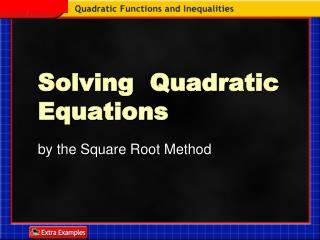DownloadDownload PresentationTélécharger la présentation- - - - - - - - - - - - - - - - - - - - - - - - - - - E N D - - - - - - - - - - - - - - - - - - - - - - - - - - -
##### Presentation Transcript

1. 10.4 Solving Quadratic Equations by the Square Root Method

2. Square Root Method • Use if there is no linear term. (i.e. B = 0) • Get the Quadratic Term on one side and the Constant on the other side. • Simply take the Square Root of Both Sides.

3. Square Root Method Be sure to give both the positive and negative answers!

4. Square Root Method Be sure to give both the positive and negative answers!

5. Solve by using the Square Root Property. Original equation Factor the perfect square trinomial. Square Root Property Subtract 7 from each side. Write as two equations. or Solve each equation. Example 4-1a Answer:The solution set is {–15, 1}.

6. Solve by using the Square Root Property. Example 4-1b Answer:{3, 13}

7. Solve by using the Square Root Property. Original equation Factor the perfect square trinomial. Square Root Property Add 5 to each side. Write as two equations. or Use a calculator. Example 4-2a

8. Example 4-6b Classwork/Homework